# CAIIB ABFM Module C Unit 3 : Other Non-DCF Valuation Models

## CAIIB Paper 3 ABFM Module C Unit 3 : Other Non-DCF Valuation Models (New Syllabus)

IIBF has released the New Syllabus Exam Pattern for CAIIB Exam 2023. Following the format of the current exam, CAIIB 2023 will have now four papers. The CAIIB Paper 3 (ADVANCED CONCEPTS OF FINANCIAL MANAGEMENT) includes an important topic called “Other Non-DCF Valuation Models”. Every candidate who are appearing for the CAIIB Certification Examination 2023 must understand each unit included in the syllabus.

In this article, we are going to cover all the necessary details of CAIIB Paper 3 (ABFM) Module C (Valuation, Mergers & Acquisitions) Unit 3 : Other Non-DCF Valuation Models, Aspirants must go through this article to better understand the topic, Other Non-DCF Valuation Models and practice using our Online Mock Test Series to strengthen their knowledge of Other Non-DCF Valuation Models. Unit 3 : Other Non-DCF Valuation Models

### Introduction

Non-DCF methods for valuation are as follows:-

• Relative Valuation Model
• Equity Valuation Multiples Model
• Enterprise Value Multiples Model
• Book Value Approach Model
• Stock and Debt Approach Model

### Relative Valuation Model

A relative valuation places an asset’s worth in comparison to the prices at which other, comparable assets are selling on the market, at the moment.

#### Steps Involved in Relative Valuation

Conducting Research on the Concerned Organisation

• You need to perform a comprehensive analysis of the subject company, which is the company that will ultimately be evaluated. This analysis should focus on the subject company’s position within its industry and its financial standing.

• Following the analysis of the subject firm, the next stage is to choose other businesses that are comparable to the original company in terms of the types of industries they specialise in, the kind of customers they cater to, the scope of their operations, and so on.

Determining the Multiples Used in Valuation: They can be organised into the following two major groups:

• Stock valuation multiples, such as the price-earnings ratio, the price-book value ratio, and the price sales ratio, and
• Enterprise valuation multiples, (EV-EBITDA ratio, EV-FCFF ratio, EV-book value ratio, and EV-sales ratio).

Figuring Out the Valuation Multiples for the Other Comparable Companies:

• Calculate the valuation multiples for each of the similar companies by using the observed financial characteristics and values of the companies being compared.

To illustrate this point, let’s imagine that there are two similar businesses, A and B, and their respective financial data is as follows: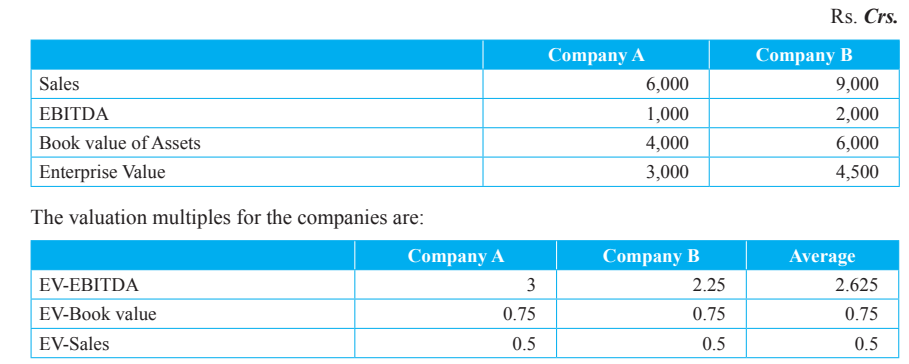Establishing a Value for the Concerned Business

• It is possible to evaluate the subject company if one considers the valuation multiples that have been observed for comparable companies.
• You can accomplish this in a straightforward manner by applying the average multiples of the comparable companies to the pertinent financial attributes of the subject company.

EXAMPLE:

The following financial information is available for company M, an unlisted pharmaceutical company, which is being valued.

• EBITDA: Rs. 500 crore
• Book value of assets: Rs. 2,000 crore
• Sales: Rs. 5,500 crore

The pharmaceutical businesses E, F, and G have been identified to be comparable to company M based on an analysis of a number of other publicly traded pharmaceutical companies.

The following information regarding the financial health of these companies is available: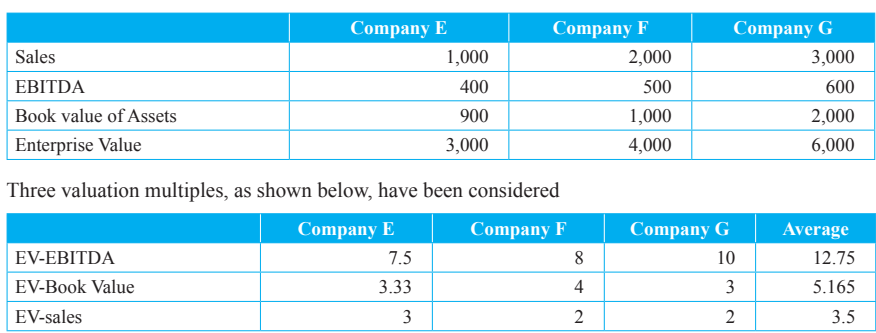The following estimations of Company M’s enterprise value can be derived by applying the average multiples to the company’s financial figures:

A simple arithmetic average of the three estimates of EV is:  =

(6,375 + 10,330 + 19,250) / 3 = Rs. 11,985 crore

### Equity Valuation Multiples Model

#### P/E Multiple:

• P/E multiple = Market price per share/ Earnings per share.
• one way to express the price-earnings multiple is as follows: P0 /E1 where P0 is the current market price per share and E1 is the expected earnings per share a year from now.

Fundamental Determinants of the P/E MultipleWhere (1 – b) is the dividend payout ratio, r is the cost of equity, ROE is the return on equity, and b is the plough back ratio.

Illustration: V & S Company’s Return on Equity is 20 percent and its r is 15 percent. Company’s dividend payout ratio is 0.3 and its retention ratio 0.7. Find its P/E Multiple.

Solution:

V & S Company’s P/E multiple is: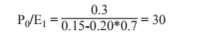#### P/B Multiple:

• P/B multiple = Market price per share/ Book value per share.
• Book value per share (B) = (Shareholders funds-Preference capital)/ Number of outstanding equity shares

Fundamental Determinants of the P/B Multiple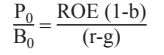Where ROE is the return on equity, g is the growth rate, (1 – b) is the dividend payout multiple, and r is the rate of return required by equity investors.

Illustration: Vitthal Limited’s ROE is 25 percent and its r is 18 percent. Vitthal’s dividend payout ratio is 0.5 and its g is 15 percent. From a fundamental point of view, find Vitthal’s P/B multiple.

Solution: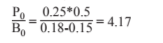#### P/S Multiple:

The price-to-sales multiple, is determined by dividing the current stock price of a firm by the revenue per share that the company has generated over the course of the most recent twelve months.

It is generally accepted that a P/S multiple of 1.0 can serve as a benchmark for comparison purposes across all businesses. Therefore, equities that are now trading at a P/S ratio that is far lower than 1.0,such as 0.5,  may be considered to be excellent deals.

Basic P/S Determinants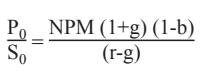Where NPM is the net profit margin ratio, g is the growth rate, (1 – b) is the dividend pay-out multiple, and r is the rate of return required by equity investors.

Illustration:

VLC Limited has a NPM of 10 percent and a growth rate 14 percent. VLC dividend pay-out ratio (1 – b) is 0.4 and its r is 0.19. Find VLC P/S multiple.

Solution: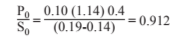#### PEG

• PEG Ratio is defined as the ratio of price to earnings divided by the anticipated annual growth rate in earnings per share.
• PEG ratio = PE ratio/Expected growth rate
• The multiple of the P/B to the ROE, is known as the value ratio.

Value Ratio =  P/B / ROE

• The multiple of P/S to NPM is referred to as PSM. PSM = P/S / NPM
• the company is undervalued if the PEG multiple is less than 1, whereas it would appear that the stock is overvalued if the PEG multiple is greater than 1.

#### Relative PE Ratio:

• A company’s price earnings ratio is measured in relation to the average for the market using relative price earnings ratios.
• You can calculate it by dividing the current PE ratio of a company by the average PE ratio for the market: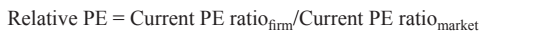### Enterprise Value Multiples Model

Enterprise value multiples put the emphasis on the value of the business itself, in contrast to equity multiples, which place the emphasis on the value of the equity. Typically, some measure of earnings, assets, or sales contributes to the determination of the enterprise value.

The following are some examples of enterprise value multiples that are regularly used:

• EV/EBITDA multiple
• EV/EBIT multiple
• EV/FCFF multiple
• EV/BV multiple
• EV/Sales multiple

#### EV to EBITDA Multiple

It stands for Enterprise value (EV)/ Earning before interest, taxes, depreciation, and amortization (EBITDA).

EV/EBITDA= (Market Value of Equity + Market Value of Debt – Cash) / EBITDA

Basic Determinants:Where ROIC stands for return on invested capital, g is for growth rate, DA stands for depreciation and amortisation charges as a percent of EBITDA, t stands for tax rate, and WACC stands for weighted average cost of capital.

Illustration: JJ Company’s ROIC is 20 percent and its g is 11 percent. JJ’s DA is 9 percent and its tax rate is 30 percent.  JJ’s WACC is 13 percent. Calculate JJ’s EV/EBITDA.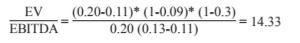Relation between Free cash flow to firm and EBITDA

• FCFF = EBIT (1 – T) + Depreciation and amortization – Capital expenditure (CAPEX) – Change in net working capital (NWC)
• EBITDA = EBIT + Depreciation and amortization
• FCFF = EBITDA – (T x EBIT) – CAPEX – change in NWC

EV to EBIT Multiple

It stands for Enterprise value (EV)/ Earnings before interest and taxes (EBIT)

Basic Determinants: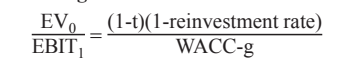Where It is the tax rate, WACC is the weighted average cost of capital, and g is the growth rate.

Illustration: ABC Company has a tax rate of 30 percent and a reinvestment rate of 70 percent. ABC WACC is 15 percent and growth rate is 12 percent. Calculate EV/EBIT.

Solution: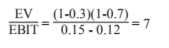EV to FCFF Multiple

It stands for Enterprise value (EV)/ Free cash flow to firm (FCFF)

Basic Determinants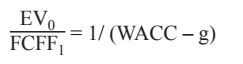Where WACC is the weighted average cost of capital and g is the growth rate.

Illustration: XYZ Limited’s WACC is 16 percent and its g is 11 percent. Calculate EV/FCFF.

Solution:

EV0/FCFF1 = 1/(0.16-0.11) = 20

#### EV to BV Multiple

It stands for Enterprise value (EV)/ Book value of assets (BV)

Basic Determinants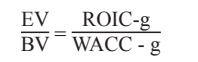Where ROIC is the return on invested capital, g is the growth rate, and WACC is the weighted average cost of capital.

Illustration: Vivek Company has an ROIC of 18 percent, growth rate of 11 percent, and WACC of 13 percent. Calculate EV/BV.

Solution:

EV/BV= (0.18-0.11)/ (0.13 – 0.11) = 3.5

### EV to Sales Multiple

• It stands for Enterprise value(EV)/Sales
• Basic Determinants

EV/S=[After tax operating margin(1+g)(1-reinvestment rate)]/ (WACC – g)

where g is the growth rate and WACC is the weighted average cost of capital.

Illustration: Planned Limited’s after-tax operating margin is 11 percent and growth rate is 9 percent. Its reinvestment rate is 70 percent and the WACC is 14 percent. Calculate EV/S.

Solution:

EV/S = [0.11(1+0.09)(1-0.70)]/ (0.14-0.09) = 0.72

### Book Value Approach Model

• Relying just on the data shown in a company’s balance sheet is the least complicated method for determining the company’s market value. Values that are based on uncertain projections of the future are considered by some to be a less accurate assessment of value than values that are based on book values that are found on a balance sheet.
• Company’s balance sheet able to produce a trustworthy assessment of the value of the firm’s assets and equity.
• Book value is a good proxy for real worth when applied to a tangible asset that has no growth potential, very few growth opportunities, or no chances at all for producing greater returns. Book value and true worth will be very different for an organisation that has large chances for growth and the possibility of producing returns that are significantly higher than average.
• The degree of precision that may be achieved using the book value technique is directly proportional to the degree to which the net book values of the assets accurately represent their fair market values.

There are three potential causes for a discrepancy between book prices and market values:

• The difference between the asset’s book value and its current value will widen as a result of inflation.
• As a result of advancements in technology, certain assets become obsolete and useless even before they have been completely written off in the books as having been depreciated.
• On the balance sheet, organisational capital does not appear, despite the fact that it is a very important asset.

#### Book Value Revision to Account for Replacement Cost

• The earning power of an asset might not be related to the book value of the asset, particularly if the asset is old, it is likely to be related to the current cost of replacing the asset. Therefore, current replacement costs may be used in place of the asset’s book value.
• Cash and Cash Equivalents: The value of money does not change under any circumstances. As a result, assigning a value to it is not problematic in any way.
• Receivables: In most cases, the value of receivables is determined by their face value. When the creditworthiness of the debtors is in question, it is prudent to set aside money to cover possible defaulted debts.

Stocks in hand:

• Raw materials, work-in-process, and finished goods are the three distinct types of inventory that can be distinguished from one another. The most recent cost of acquisition may be used to determine the worth of raw materials.
• Either the cost of work-in-process (which includes the cost of raw materials in addition to the cost of processing) or the selling price can be used as a lens through which to analyse work-in-process (selling price of the final product less expenses to be incurred in translating work-in-process into sales).
• Inventory of finished goods is valued by deducting the expenses that will be incurred in the process of packaging, processing, transporting, selling, and collecting receivables, from the sale price that can be expected to be realised in the normal course of business.

Other Current Assets:

• Deposits, prepaid expenses, and accruals are examples of other types of current assets that are recognized at their book value.

Non-Current Assets:

• Land, buildings and other civil works, as well as machinery and plant, make up the majority of a company’s tangible fixed assets. The value of land is determined as if it were uninhabited and on the market.
• It is possible to value buildings and civil works by subtracting the amount of physical depreciation and deterioration from the cost of replacement.
• It is possible to determine the worth of plant and machinery by calculating the market price of comparable (used) assets and adding the cost of transportation and installation to that figure.

Intangible Fixed Assets:

• Patents, software, copyrights, mining leases, licences, spectrum etc. are examples of Intangible assets. They can be valued at their fair market price.

Non-operating Assets:

• The assets of an organisation that are not essential to its day-to-day operations are referred to collectively as “non-operating assets.”
• Financial securities, surplus land, and disused structures are the three types of non-operating assets that are most frequently encountered. These assets are evaluated based on their true value in the market.

#### Adjusting Book Values to Reflect Liquidation Values

• Finding out how much cash the assets listed on a company’s balance sheet could bring in if the company were immediately put into liquidation is the method that provides the most accurate approximation of the fair market value of those assets.
• If there is a healthy secondary market for the assets, then the liquidation values will be the same as the prices on the secondary market. Nevertheless, there aren’t any active secondary markets for the majority of business assets.
• The fact that the liquidation value approach pays no attention to organisational capital is its most significant shortcoming. The company is valued not as a going concern but rather as a collection of assets that can be sold separately from one another.

Problems with Asset-Based Valuation

The asset-based valuation approach, in essence, makes an attempt to recast the balance sheet by (a) determining the assets and liabilities that are included on the balance sheet based on their most recent values in the market and (b) determining which assets and liabilities are not included on the balance sheet and assigning a market value to each of those assets and liabilities.

However, despite its apparent ease, it actually raises a number of very challenging issues:

• The assets that are included on the balance sheet might not be actively traded, which means that it might be difficult to obtain accurate market values for them.
• Even if the information is available, market values may not accurately reflect the true value of the asset due to inefficiencies in the market.
• It is possible that the cost of an asset’s curren replacement or its selling price (its liquidation value) does not accurately reflect the value that the asset contributes when used in a particular going concern.
• Intangible assets are typically what are considered omitted assets. It can be difficult to recognise and assess their worth.
• Even if individual assets can be located and valued, it is likely that the total of the individual values of all identified assets will not equal the value of the assets as a whole. It is difficult to put a price on the asset known as “synergy.” The question that needs to be answered in order to properly value the company is how much its individual assets are worth when added together.

### Stock And Debt Approach

• When a company’s securities are traded on a public exchange, the value of the company can be determined by simply adding up the current market value of all of the company’s outstanding securities. Appraisers of properties use a straightforward methodology that they refer to as the stock and debt approach.
• Another name for this strategy is the market-based approach.